# 1 Introduction

Biodiversity in any sampled area depends on three components

1. The total number of individuals
2. The relative abundances of species
3. The spatial distribution of species

Changes of each component result in specific signatures with respect to the change of non-spatial and spatial biodiversity patterns (see vignette Analysis of biodiversity patterns). Therefore, biodiversity patterns can be assessed to investigate the scale-dependent effects of biodiversity drivers, e.g. habitat loss, climate change etc. on the three biodiversity components.

In this vignette we simulate independent variations of each of the three components and demonstrate how rarefaction and species-accumulation curves can be applied to diagnose changes in biodiversity components.

For this purpose we first simulate a reference community. We assume a random distribution of species in sapce, so we can use the function sim_poisson_community:

library(mobsim)

S0 <- 100
N0 <- 1000
cv0 <- 0.5

sim_ref <- sim_poisson_community(s_pool = S0, n_sim = N0, sad_type = "lnorm",
sad_coef = list("cv_abund" = cv0), fix_s_sim = T)
plot(sim_ref)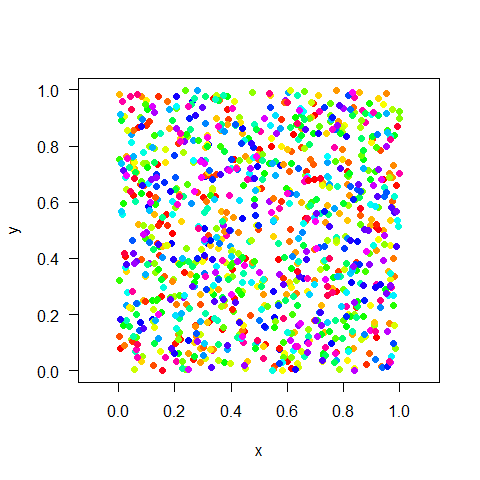# 2 Change in total number of individuals

First we vary the total number of individuals. For this purpose we simulate a second community with just half of the individuals.

sim_lower_N <-  sim_poisson_community(s_pool = S0, n_sim = N0/2, sad_type = "lnorm",
sad_coef = list("cv_abund" = cv0))

Then we evaluate the rarefaction and species-accumulation curve for both communities

curve_ref    <- spec_sample_curve(sim_ref)
curve_lower_N <- spec_sample_curve(sim_lower_N)

par(mfrow = c(1,2))
plot(spec_rarefied ~ n, data = curve_ref, type = "l", main = "Rarefaction curve",
xlab = "No. of individuals", ylab = "No. of species" )
lines(spec_rarefied ~ n, data = curve_lower_N, col = "red")

plot(spec_accum ~ n, data = curve_ref, type = "l", main = "Species accumulation curve",
xlab = "No. of individuals", ylab = "No. of species" )
lines(spec_accum ~ n, data = curve_lower_N, col = "red")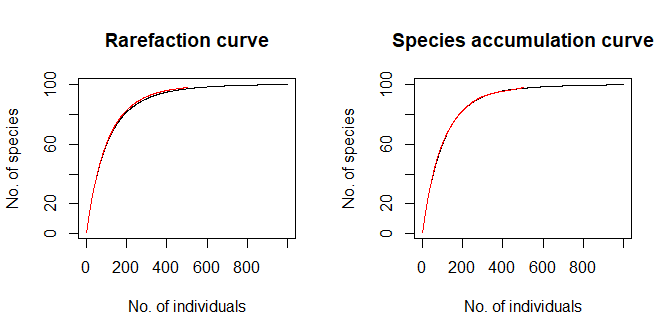Due to the stochasticity of simulations the curves of the reference and the changed community slightly differ, but on average random losses of individuals are indicated by overlapping curves, which end at different numbers of individuals.

# 3 Change in the abundance distribution

The second simulated change represents a reduction of the evenness of the species abundance distribution, i.e. there are more very rare and more very common species than in the reference community. In mobsim there is the argument cv_abund in the simulation functions, which is the coefficient of variation (cv) of species abundances. The higher the cv the lower the evenness and vice versa. We also use the argument fix_s_local= T to simulate a community with exactly S0 species.

sim_uneven <-  sim_poisson_community(s_pool = S0, n_sim = N0, sad_type = "lnorm",
sad_coef = list("cv_abund" = cv0*4),
fix_s_sim = T)
curve_uneven <- spec_sample_curve(sim_uneven)

par(mfrow = c(1,2))
plot(spec_rarefied ~ n, data = curve_ref, type = "l", main = "Rarefaction curve",
xlab = "No. of individuals", ylab = "No. of species" )
lines(spec_rarefied ~ n, data = curve_uneven, col = "red")

plot(spec_accum ~ n, data = curve_ref, type = "l", main = "Species accumulation curve",
xlab = "No. of individuals", ylab = "No. of species" )
lines(spec_accum ~ n, data = curve_uneven, col = "red")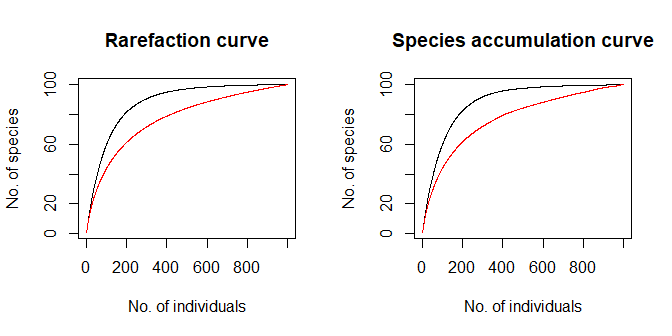As we see changes in the abundance distributions without changes in total species richness are indicated by changing slopes and shapes of the curves.

# 4 Change in spatial distributions

Finally we change the spatial patterns of species, by simulating clumpoing of conspecific individuals using a Thomas process model instead of a Poisson

sim_clumped <-  sim_thomas_community(s_pool = S0, n_sim = N0, sad_type = "lnorm",
sad_coef = list("cv_abund" = cv0),
fix_s_sim = T,
sigma = 0.05)

par(mfrow = c(1,2))
plot(sim_ref)
plot(sim_clumped)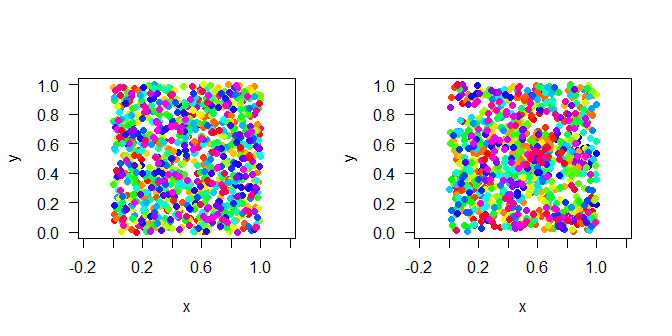As before we compare the rarefaction and accumulation curves:

curve_clumped<- spec_sample_curve(sim_clumped)

par(mfrow = c(1,2))
plot(spec_rarefied ~ n, data = curve_ref, type = "l", main = "Rarefaction curve",
xlab = "No. of individuals", ylab = "No. of species" )
lines(spec_rarefied ~ n, data = curve_clumped, col = "red")

plot(spec_accum ~ n, data = curve_ref, type = "l", main = "Species accumulation curve",
xlab = "No. of individuals", ylab = "No. of species" )
lines(spec_accum ~ n, data = curve_clumped, col = "red")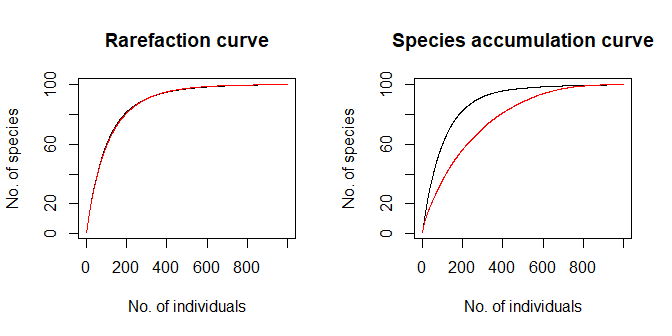When there is only a change in spatial distribution the rarefaction curve does not change, because it only depends on species abundances, but not on spatial distributions (see vignette Analysis of biodiversity patterns). In contrast the species-accumulation curves indicates higher intraspecific clumping (also called aggregation) by a lower slope and lower expected species richness for the same number of sampled individuals.